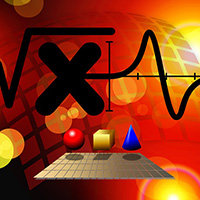Global

Mathematics Experts

physico-mathematical sciences
Saratov State Technical University
Russian Federation

### Biography

2007 - defended a thesis for the degree of candidate of physical and mathematical sciences "Mathematical models and algorithms for the analysis of special photographic images (digital and halogen silver)".

#### Research Interest

physico-mathematical sciences